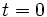# Eternal solution

## Definition

### Given data

A differential equation with one independent variable$t$ (called the time parameter) and a vector-valued dependent variable, along with an initial value condition at$t=0$

### Definition

A solution function$f$ for the differential equation is termed an eternal solution if$f$ is well-defined for all$t$.

Clearly, if we find an eternal solution, we have also found solutions for all open intervals inside$\R$, simply obtained by restricting the eternal solution.

Moreover, if a uniqueness theorem is applicable to the differential equation subject to the initial value conditions, then we further conclude that any solution overan interval is in fact the restriction to that interval of the eternal solution.

## Relation with other solution properties

### Weaker properties

• Immortal solution is a solution that can be defined for all positive$t$
• Ancient solution is a solution that can be defined for all negative$t$